This post will guide you how to use Google Sheets AVERAGEIF function with syntax and examples in Google Spreadsheet.

## Description

The Google Sheets AVERAGEIF function returns the average of all numbers in a range of cells that meet a given criteria.

The AVERAGEIF function is a build-in function in Google Sheets and it is categorized as a Statistical Function.

## Syntax

The syntax of the AVERAGEIF function is as below:

`= AVERAGEIF (range, criteria, [average_range])`

Where the AVERAGEIF function arguments are:

• `Range `-This is a required argument.  The range of cells that you want to apply the criteria to average
• `Criteria `– This is a required argument.  The criteria used to define which cells are averaged
• `Average_range`This is an optional argument.  The average range is actually to average. If it is omitted, the function will use Range values to average.

## Google Sheets AVERAGEIF Function Example

The below examples will show you how to use Google Sheets AVERAGEIF Function to return the average of all numbers in a range of cells based on a given criteria.

#1 To get the average of all numbers in average range B1:B5 and meet the criteria that equal to “2022” in range A1:A5, and the, just using the following Google Sheets formula:

`=AVERAGEIF(A1:A5,2022,B1:B5)`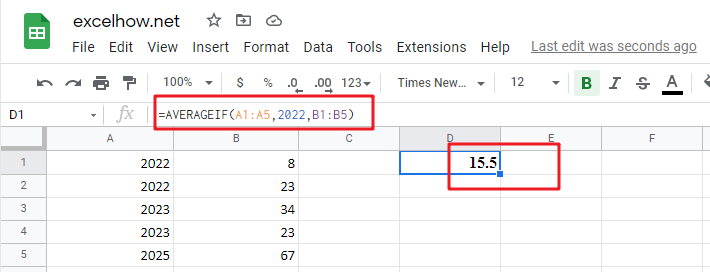## Calculating Average Of The Numbers in Google Sheets

Are you weary of investing a lot of time and effort in manually calculating the average of the numbers by including or excluding 0 and calculating the average of the top 3 scores? Then congratulations because you have just landed on the right article.

In this article, you will get to know the smarter ways to do these cumbersome tasks in a matter of seconds.

So without any delay, let’s dive into the article,

## Average Of The Numbers By Including 0

### General Formula

Use the formula below to calculate the average of a set of numbers in Google Sheets:

`=AVERAGE(range)`

Explanations for Syntax:

•  AVERAGE: In Google Sheets, the AVERAGE Function can calculate the arithmetic mean of a set of numbers.
• Range: This is the input value from your worksheet.
• Comma symbol (,): It acts as a separator that helps to separate a list of values.
• Parenthesis (): The main Function of this symbol is to group the elements.

### Explanation

Use the AVERAGE function to calculate the average of a set of numbers.

In the example, the formula in E3 is:

`=AVERAGE(A3:C3)`

###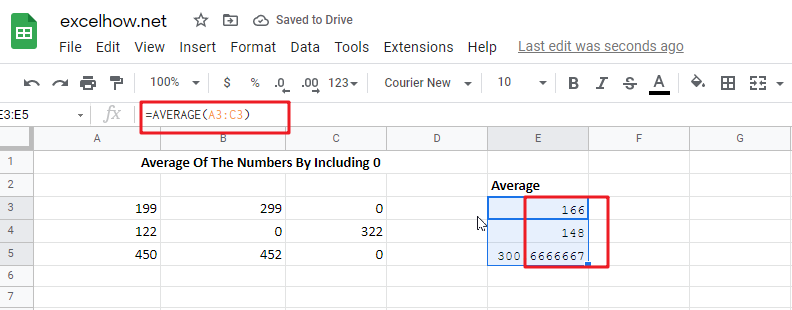which is then copied down in the table.

AVERAGE is a built-in function in Google Sheets in which you supply a range of cells to average, and this function returns the result. In cases where cells are not adjacent, you can also provide individual arguments to the Function.

The AVERAGE function automatically ignores all the blank text and cells, but zero values are included.

## Average Of The Numbers By Ignoring 0

### General Formula

The formula below will assist you in calculating the average of a number that excludes zero:

`=AVERAGEIF(range,"<>0")`

Explanations for Syntax:

• AVERAGEIF: This Function returns the average of a set of input values that satisfy a single condition or criteria. Learn more about the AVERAGEIF Function.
• Range: This is the input value from your worksheet.
• Comma symbol (,): It acts as a separator that helps to separate a list of values.
• Parenthesis (): The main Function of this symbol is to group the elements.
• Operator (>): The supplied criteria is “>0,” which means “not equal to zero.”

### Explanation

The AVERAGEIF function computes the average of a set of numbers while excluding or ignoring zero values. In the example, the formula in E9 is:

`=AVERAGEIF(A9:C9,"<>0")`

###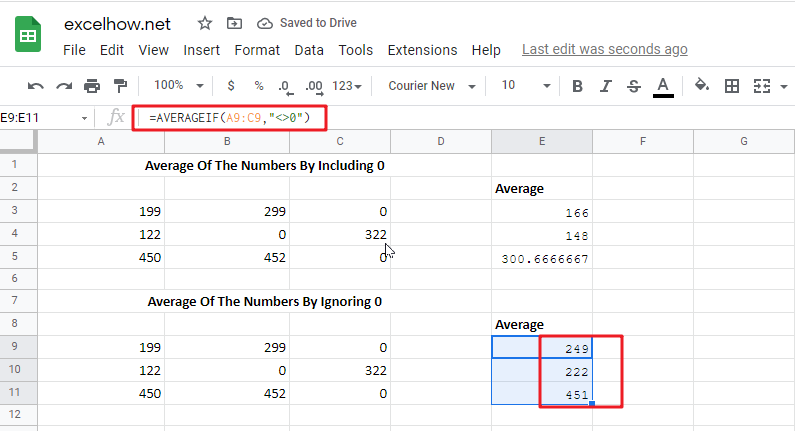The formula in E3 in the example is based on the AVERAGE Function:

`=AVERAGE(A3:C3)`

Because (129+299+0)/ 3 = 166, the answer is 166.

To remove the zero from the calculated average, the formula in E10 employs the AVERAGEIF function, as shown below:

`=AVERAGEIF(A10:C10,"<>0") / returns 249`

The provided criterion is “>0,” which means “not equal to zero”.

### Data with no values

Because the AVERAGE, AVERAGEIF, and AVERAGEIFS functions all ignore blank cells (and cells with text values), there isn’t any need to provide criteria to filter out empty cells.

## Average Of The Top 3 Numbers

### General Formula

The formula below will assist you in calculating the average of the top 3 numbers:

`=ArrayFormula(AVERAGE(LARGE(range,{1,2,3})))`

Explanations for Syntax:

• AVERAGE: In Google Sheets, the AVERAGE Function can calculate the arithmetic mean of a set of numbers.
• Range: This is the input value from your worksheet.
• Comma symbol (,): It acts as a separator that helps to separate a list of values.
• Parenthesis (): The main Function of this symbol is to group the elements.
• LARGE: This Function returns the Nth largest value from the given range of data. Learn more about the LARGE Function.
• Values: This is the range of cells that contain values.

### Explanation

To average the top three values in a data set, use a formula based on the LARGE and AVERAGE functions. In this example, the formula in G15 is mentioned below:

`=ArrayFormula(AVERAGE(LARGE(A15:E15,{1,2,3})))`

###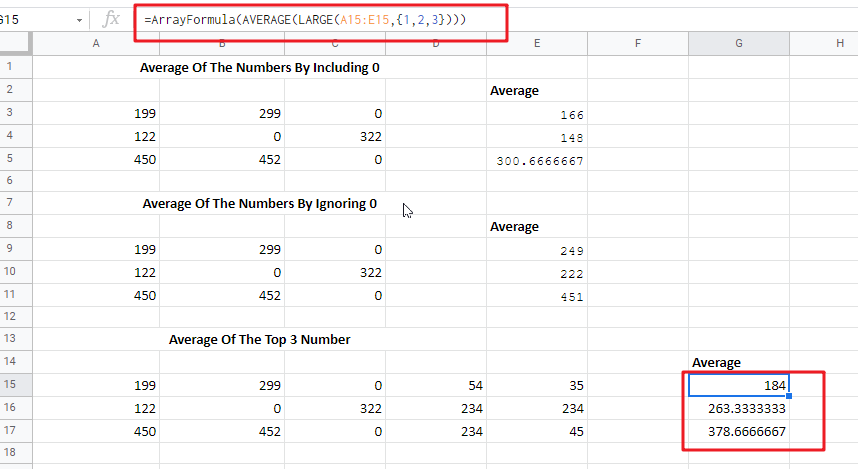The LARGE function is used to find the top nth value in a set of numbers. For example, the LARGE(A15:E15,{1,2,3}) will return the highest value, LARGE(A15:E15,2)will return the second-highest value, and so on:

```=LARGE(A15:E15,1)/First largest value
=LARGE(A15:E15,2)/ 2nd largest value
=LARGE(A15:E15,3)/ 2nd largest value```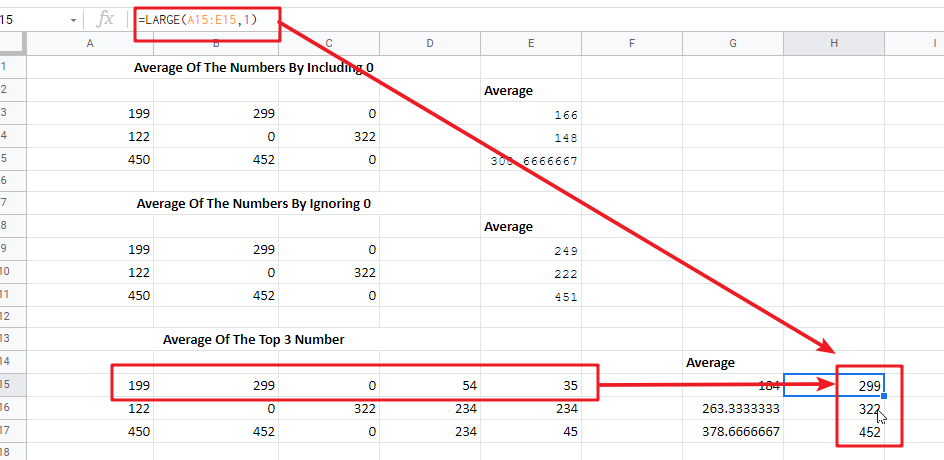In this case, we are requesting multiple values by passing an array constant 1,2,3 into LARGE as the second argument. This causes LARGE to return an array of the top three values.

`= LARGE(A15:E15,{1,2,3})`

returns an array similar to this:

`{299,199,54}`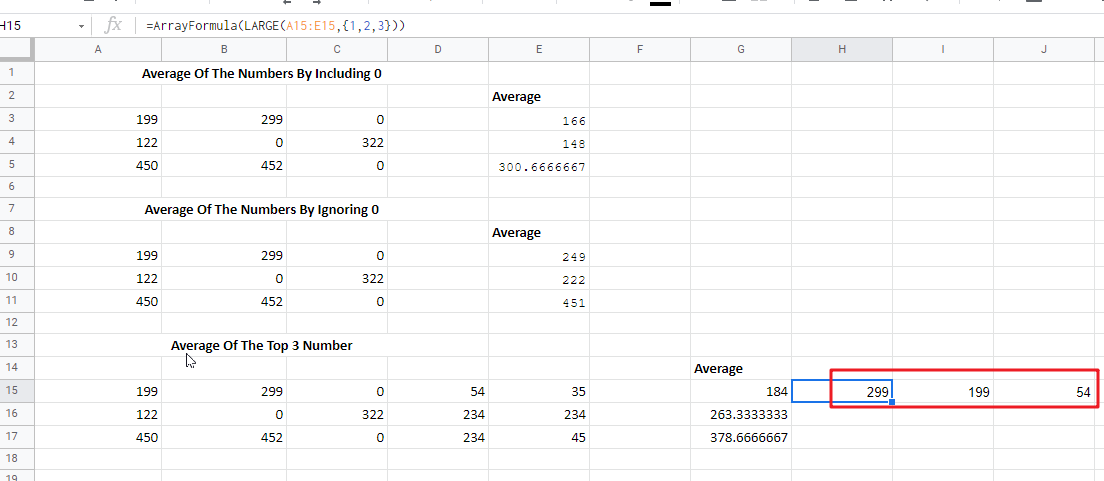This array is passed directly to the AVERAGE function:

`=AVERAGE(299,199,54) / returns 184`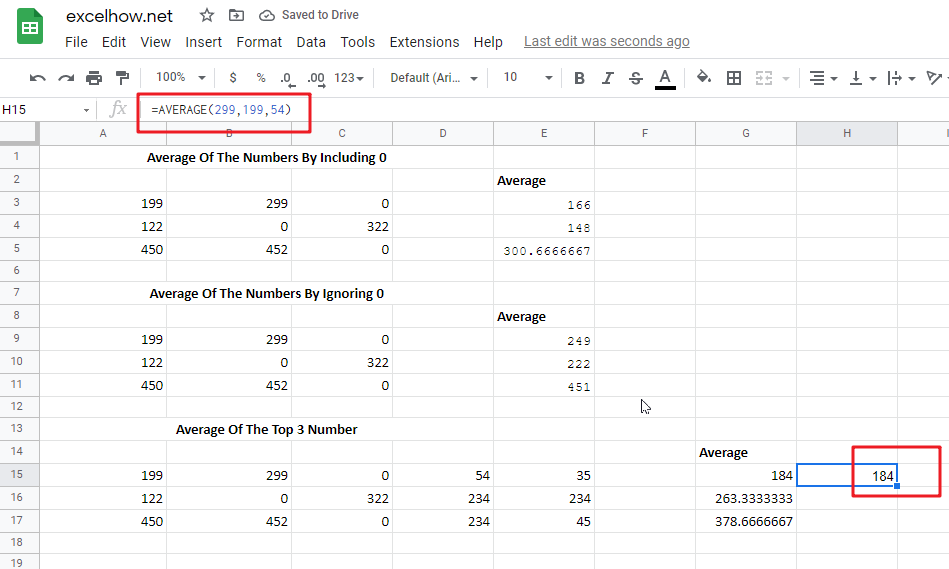The AVERAGE Function then returns the average of these values.

Because the AVERAGE Function can handle arrays natively, there is no need to enter this formula with control + shift + enter.